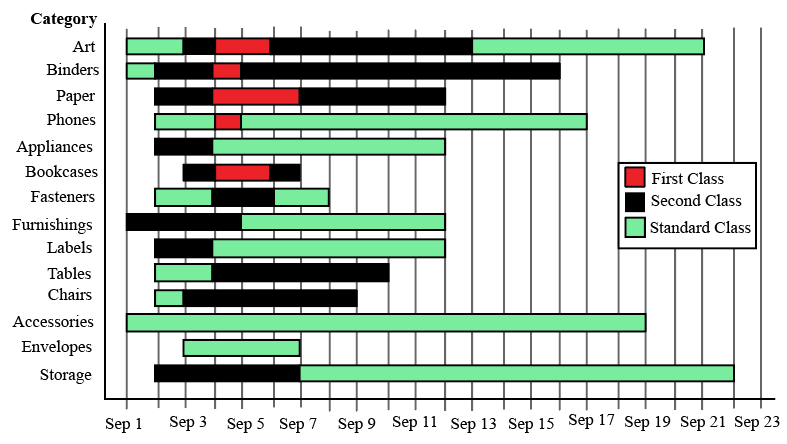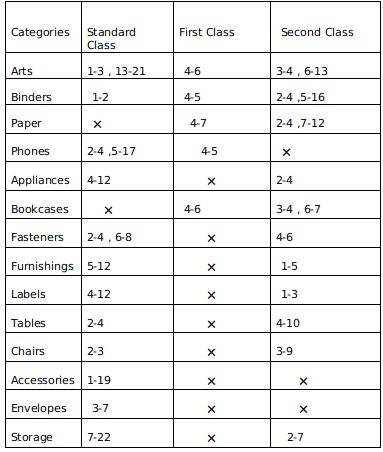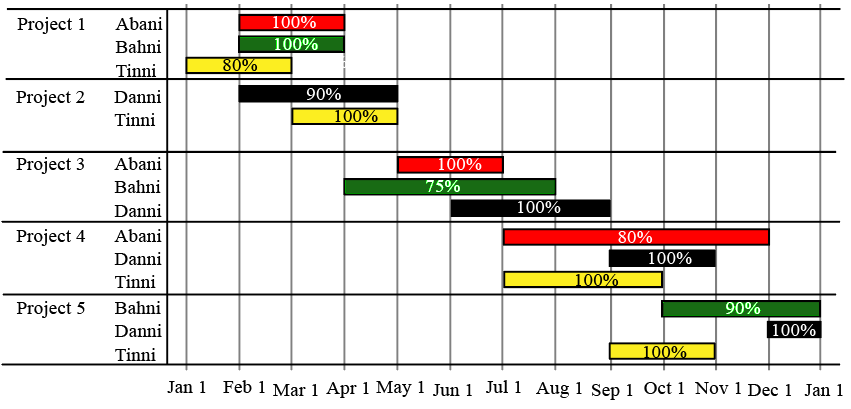# DI Questions for CAT

0
492Data Interpretation (DI) is one of the most important topics in LRDI section of the CAT. For many aspirants, LRDI has become the deciding section in the CAT. Hence, it is extremely important that you take this section seriously. You can check out these DI CAT Previous year questions. Practice a good number of Sets in the CAT DI questions. Also, over the past few years most of the sets that appear in the CAT, are a mix of LR and DI concepts. In this article, we will look into some important DI Questions for CAT. These are a good source for practice; If you want to practice these questions, you can download this CAT DI Questions PDF below, which is completely Free.

InstructionsThe different bars in the diagram above provide information about different orders in various categories (Art, Binders, ….) that were booked in the first two weeks of September of a store for one client. The colour and pattern of a bar denotes the ship mode (First Class / Second Class / Standard Class). The left end point of a bar indicates the booking day of the order, while the right end point indicates the dispatch day of the order. The difference between the dispatch day and the booking day (measured in terms of the number of days) is called the processing time of the order. For the same category, an order is considered for booking only after the previous order of the same category is dispatched. No two consecutive orders of the same category had identical ship mode during this period.

For example, there were only two orders in the furnishing category during this period. The first one was shipped in the Second Class. It was booked on Sep 1 and dispatched on Sep 5. The second order was shipped in the Standard class. It was booked on Sep 5 (although the order might have been placed before that) and dispatched on Sep 12. So the processing times were 4 and 7 days respectively for these orders.

Question 1: How many days between Sep 1 and Sep 14 (both inclusive) had no booking from this client considering all the above categories?

Solution:

Accumulating all the data :
We get the following table :Note a-b : represents the duration where a is the day when order is booked and b is the day when it is dispatched .
Now No booking days from the table are : September 8,9,10,11,12 and 14.
So a total of 6 days .

Question 2: What was the average processing time of all orders in the categories which had only one type of ship mode?

Solution:

Accumulating all the data :
We get the following table :Note a-b : represents the duration where a is the day when order is booked and b is the day when it is dispatched .
Now Envelopes and Accessories has only 1 ship mode i.e Standard class .
So therefore processing days for envelopes = 7-3 =4
and processing days for accessories = 19-1 =18
Therefore average = $\frac{\left(18+4\right)}{2}=11$

Question 3: The sequence of categories — Art, Binders, Paper and Phones — in decreasing order of average processing time of their orders in this period is:

a) Art, Binders, Paper, Phones

b) Phones, Art, Binders, Paper

c) Phones, Binders, Art, Paper

d) Paper, Binders, Art, Phones

Solution:

Accumulating all the data :
We get the following table :Note a-b : represents the duration where a is the day when order is booked and b is the day when it is dispatched .

Now taking average processing time per order for the above mentioned categories we get :
Art =$\frac{2+8+2+1+7}{5}\ \ =4$
Binders =$\frac{1+1+11+2}{4}\ \ =3.75$
Papers = $\frac{3+2+5}{3}\ \ =3.33$
Phones =$\frac{2+12+1}{3}\ \ =5$
So in decreasing order we get Phones , Art ,Binder , Paper.

Question 4: Approximately what percentage of orders had a processing time of one day during the period Sep 1 to Sep 22 (both dates inclusive)?

a) 22%

b) 16%

c) 20%

d) 25%

Solution:

Accumulating all the data :
We get the following table :Note a-b : represents the duration where a is the day when order is booked and b is the day when it is dispatched .

Now from the table we observe that the total number of orders are 35 and 7 orders have a processing time of 1 unit
The 7 orders are : Arts Standard class,Binders First class and standard class, Phones First class, Bookcases second class ( 2 orders)  and Chairs standard class.
So the percentage = $\frac{7}{35}\times\ 100\ =\ 20$

InstructionsThe figure above shows the schedule of four employees – Abani, Bahni, Danni, and Tinni – whom Dhoni supervised in 2020. Altogether there were five projects which started and concluded in 2020 in which they were involved. For each of these projects and for each employee, the starting day was at the beginning of a month and the concluding day was the end of a month, and these are indicated by the left and right end points of the corresponding horizontal bars. The number within each bar indicates the percentage of assigned work completed by the employee for that project, as assessed by Dhoni.

For each employee, his/her total project-month (in 2020) is the sum of the number of months (s)he worked across the five projects, while his/her annual completion index is the weightage average of the completion percentage assigned from the different projects, with the weights being the corresponding number of months (s)he worked in these projects. For each project, the total employee-month is the sum of the number of months four employees worked in this project, while its completion index is the weightage average of the completion percentage assigned for the employees who worked in this project, with the weights being the corresponding number of months they worked in this project.

Question 5: Which of the following statements is/are true?
I: The total project-month was the same for the four employees.
II: The total employee-month was the same for the five projects.

a) Only II

b) Both I and II

c) Neither I nor II

d) Only I

Solution:

The total project month is the number of months Abani, Bahni, Danni, and Tinni individually worked for all the projects combined :

Abani – 2+2+5 = 9 months

Bahni – 2+4+3 = 9 months

Danni – 3+3+2+1 = 9 months

Tinni – 2+2+3+2 = 9 months.

The total employee month for all the five projects is the sum of the total employee-month is the sum of the number of months four employees worked in this project.

Project -1 = 2+2+2 = 6 months

Project -2 = 3+2 = 5 months

Project – 3 = 2+4+3 = 9 months.

Project – 4 = 5+2+3 = 10 months.

Project – 5 = 3+1+2 = 6 months.

Only statement 1 is true.

Question 6: Which employees did not work in multiple projects for any of the months in 2020?

a) Only Abani, Bahni and Danni

b) Only Abani and Bahni

c) All four of them

d) Only Tinni

Solution:

Abani, Banni, and Danni did not work on multiple projects simultaneously in a month

Tinni was the only person who worked on multiple projects which are project 4 and project 5 in the month of september.

Question 7: The project duration, measured in terms of the number of months, is the time during which at least one employee worked in the project. Which of the following pairs of the projects had the same duration?

a) Project 1, Project 5

b) Project 4, Project 5

c) Project 3, Project 5

d) Project 3, Project 4

Solution:

Considering the information provided :

For project 1 : 3 months.

Project – 2: 3 months.

Project – 3: 5 months.

Project – 4: 5 months.

Project – 5: 4 months.

Among the given options option D is true which is project 3, project 4.

Question 8: The list of employees in decreasing order of annual completion index is:

a) Danni, Tinni, Bahni, Abani

b) Bahni, Abani, Tinni, Danni

c) Danni, Tinni, Abani, Bahni

d) Tinni, Danni, Abani, Bahni

Solution:

The annual  completion index for different people is :

The weightage average of the completion percentage assigned from the different projects, with the weights being the corresponding number of months (s)he worked in these projects.

For Abani :

$\frac{\left(\left(100\cdot2\right)+\left(100\cdot2\right)+\left(80\cdot5\right)\right)}{2+2+5}=\ \frac{800}{9}$

For Bahni :

$\frac{\left(\left(100\cdot2\right)+\left(75\cdot4\right)+\left(90\cdot3\right)\right)}{2+3+4}=\ \frac{770}{9}$

For Danni :

$\frac{\left(\left(90\cdot3\right)+\left(100\cdot3\right)+\left(100\cdot2\right)+\left(100\cdot1\right)\right)}{2+3+2+1}=\ \frac{870}{9}$

For Tinni :

$\frac{\left(\left(80\cdot2\right)+\left(100\cdot2\right)+\left(100\cdot3\right)+\left(100\cdot2\right)\right)}{2+2+3+2}=\ \frac{860}{9}$

The descending order for the four people is :

Danni, Tinni, Abani, Bahni.

Instructions

An ATM dispenses exactly Rs. 5000 per withdrawal using 100, 200 and 500 rupee notes. The ATM requires every customer to give her preference for one of the three denominations of notes. It then dispenses notes such that the number of notes of the customer’s preferred denomination exceeds the total number of notes of other denominations dispensed to her.

Question 9: In how many different ways can the ATM serve a customer who gives 500 rupee notes as her preference?

Solution:

It has been given that the customer gives 500 rupee notes as her preferred denomination.
Therefore, the number of 500 rupee notes dispensed must be greater than the number of the notes of other denominations dispensed.

If Rs.3500 is dispensed as 500 rupee notes (7 notes), the remaining 1500 rupees should be dispensed using Rs.100 and Rs.200 notes. The minimum number of notes of other denomination required in this case will be 8 (7*200 + 1*100). Therefore, at least Rs.4000 should be dispensed as 500 rupee notes.

Case (1):
Rs.4000 is dispensed using 500 rupee notes, 8 five hundred rupee notes will be dispensed.
The remaining 1000 rupees cannot be fully dispensed as 100 rupee notes (since 10 notes will be required).
If 800 rupees is dispensed as 100 rupee notes, then 9 notes will be required to dispense 1000 rupees (8*100+200).
Therefore, we can eliminate these 2 cases.
If 600 rupees is dispensed using 100 rupee notes, then a minimum of 8 notes will be required to dispense 1000 rupees (6*100 + 2*200). Therefore, we can eliminate this case as well.

If 400 rupees is dispensed using 100 rupee notes, then 7 notes will be required (4*100+3*200). This is a valid case.
If 200 rupees is dispensed using 100 rupee notes, then 6 notes will be required (2*100+4*200). This is a valid case.
1000 rupees can be dispensed using 5 notes of Rs.200.

Therefore, there are 3 valid cases.

Case (2):
Rs.4500 is dispensed using 500 rupee notes. 9 five hundred rupee notes will be dispensed in this case.
The remaining 500 rupees can be dispensed as 100 rupee notes ( 5 notes) or a combination of 100 rupee and 200 rupee notes.
200*a + 100*b = 500
‘a’ can take 0, 1, and 2.
Therefore, there are 3 valid cases.

Case (3):
5000 rupees is dispensed using 10 five hundred rupee notes.
There is only 1 valid case.

Total number of valid cases = 3+3+1 = 7.
Therefore, 7 is the right answer.

Question 10: If the ATM could serve only 10 customers with a stock of fifty 500 rupee notes and a sufficient number of notes of other denominations, what is the maximum number of customers among these 10 who could have given 500 rupee notes as their preferences?

Solution:

If a customer gives 500 rupee notes as her preferred denomination, the number of 500 rupee notes dispensed must be greater than the number of the notes of other denominations dispensed.

If Rs.3500 is dispensed as 500 rupee notes (7 notes), the remaining 1500 rupees should be dispensed using Rs.100 and Rs.200 notes. The minimum number of notes of other denomination required in this case will be 8 (7*200 + 1*100). Therefore, at least Rs.4000 should be dispensed as 500 rupee notes.

Case (1):
Rs.4000 is dispensed using 500 rupee notes, 8 five hundred rupee notes will be dispensed.
The remaining 1000 rupees cannot be fully dispensed as 100 rupee notes (since 10 notes will be required).
If 800 rupees is dispensed as 100 rupee notes, then 9 notes will be required to dispense 1000 rupees (8*100+200).
Therefore, we can eliminate these 2 cases.
If 600 rupees is dispensed using 100 rupee notes, then a minimum of 8 notes will be required to dispense 1000 rupees (6*100 + 2*200). Therefore, we can eliminate this case as well.

If 400 rupees is dispensed using 100 rupee notes, then 7 notes will be required (4*100+3*200). This is a valid case.
If 200 rupees is dispensed using 100 rupee notes, then 6 notes will be required (2*100+4*200). This is a valid case.
1000 rupees can be dispensed using 5 notes of Rs.200.

Therefore, there are 3 valid cases.

Case (2):
Rs.4500 is dispensed using 500 rupee notes. 9 five hundred rupee notes will be dispensed in this case.
The remaining 500 rupees can be dispensed as 100 rupee notes ( 5 notes) or a combination of 100 rupee and 200 rupee notes.
200*a + 100*b = 500
‘a’ can take 0, 1, and 2.
Therefore, there are 3 valid cases.

Case (3):
5000 rupees is dispensed using 10 five hundred rupee notes.
There is only 1 valid case.

It has been given that the ATM could serve only 10 customers with a stock of fifty 500 rupee notes. We have to find the maximum number of customers who could have given Rs.500 as their preference.

The least number of 500 rupee notes required to serve a customer who has given Rs.500 as the preference is 8. Using 50 five hundred rupee notes, we can serve [500/8] = 6 customers. Therefore, 6 is the correct answer.

Question 11: What is the maximum number of customers that the ATM can serve with a stock of fifty 500 rupee notes and a sufficient number of notes of other denominations if all the customers are to be served with at most 20 notes per withdrawal?

a) 12

b) 10

c) 13

d) 16

Solution:

It has been given that the customer has to receive 20 notes at the maximum. Also, we have restriction on the number of 500 rupee notes (fifty) but we do not have any restriction on the number of notes of other denominations. Therefore, in order to serve the maximum number of customers, we have to minimize the number of 500 rupee notes dispensed as much as possible.

If no 500 rupee note is dispensed, then a minimum of 25 notes will be required (25 200 rupee notes).
If one 500 rupee note is dispensed, then a minimum of one 100 rupee note and twenty two 200 rupee notes will be required. The total number of notes required = 1 + 1 + 22 = 24. Therefore, we can eliminate this case.

If two 500 rupee notes are dispensed, then a minimum of 20 two hundred rupee notes will be required. We can eliminate this case as well since the number of notes required is greater than 20.

If three 500 rupee notes are dispensed, then a minimum of 1 hundred rupee note and 17 two hundred rupee notes will be required. The number of notes required in this case is 3+1+17 = 21. Therefore, we can eliminate this case as well.

If four 500 rupee notes are dispensed, then a minimum of 15 two hundred rupee notes will be required. Total number of notes required in this case is 4+15 = 19 < 20. Therefore, this is a valid case.

The least number of 500 rupee notes with which we can serve a customer such that the total number of notes dispensed does not exceed 20 is 4. Therefore, a maximum of [50/4] = 12 customers can be served with 50 five hundred rupee notes and hence, option A is the right answer.